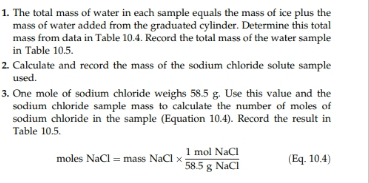# 1. The total mass of water in each sample equals the mass of ice plus themass of water added from the graduated cylinder. Determine this totalmass from data in Table 10.4. Record the total mass of the water samplein Table 10.52 Calculate and record the mass of the sodium chloride solute sampleused3. One mole of sodium chloride weighs 58.5 g. Use this value and thesodium chloride sample mass to calculate the number of moles ofsodium chloride in the sample (Equation 10.4). Record the result inTable 10.51 mol NaCI585g Naci(Eq. 10.4)moles NaCl mass NaCl x

Question
15 views
 Table 10.4 (data) B. Freezing-Point Depression Sodium Chloride Solute Ethylene Glycol Solute Mass of beaker + ice + water 111.438 g 112.337 g Freezing point of pure wate 58.650 g 53.263 g Mass of sample + container 0 C 0 C Mass of empty container 7.686 g 33.185 g Freezing point of solution 2.676 g 28.524 g
 Table 10.5 (report) Sodium Chloride Solute Ethylene Glycol Solute Total water mass Mass of solute Moles of solute ?t f Water mass in kg Solution molality K f

Use Equation to complete table 10.5 from the attached picture.help_outlineImage Transcriptionclose1. The total mass of water in each sample equals the mass of ice plus the mass of water added from the graduated cylinder. Determine this total mass from data in Table 10.4. Record the total mass of the water sample in Table 10.5 2 Calculate and record the mass of the sodium chloride solute sample used 3. One mole of sodium chloride weighs 58.5 g. Use this value and the sodium chloride sample mass to calculate the number of moles of sodium chloride in the sample (Equation 10.4). Record the result in Table 10.5 1 mol NaCI 585g Naci (Eq. 10.4) moles NaCl mass NaCl x fullscreen
check_circle

Step 1

For sodium chloride solute,

Step 2
Step 3

For ethylene glyc...

### Want to see the full answer?

See Solution

#### Want to see this answer and more?

Solutions are written by subject experts who are available 24/7. Questions are typically answered within 1 hour.*

See Solution
*Response times may vary by subject and question.
Tagged in

### Chemistry